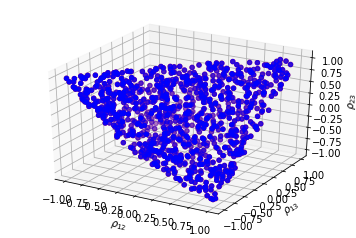# How to sample uniformly over the space of correlation matrices? The onion method

The onion method is a method that samples exactly from the uniform distribution over the set of correlation matrices, a subset of $R^{\frac{d(d-1)}{2}}$.

The method is described in Behaviour of the NORTA Method for Correlated Random Vector Generation as the Dimension Increases (Section 4).

Basically, it starts with a one-dimensional matrix and grows it iteratively by adding extra rows chosen from an appropriate distribution.

This sampling procedure can be interesting when studying how some portfolio construction algorithms behave in- and out-sample, and how do they compare each other.

### Onion method

import numpy as np
from numpy.random import beta
from numpy.random import randn
from scipy.linalg import sqrtm
from numpy.random import seed

seed(42)

def sample_unif_correlmat(dimension):
d = dimension + 1

prev_corr = np.matrix(np.ones(1))
for k in range(2, d):
# sample y = r^2 from a beta distribution
# with alpha_1 = (k-1)/2 and alpha_2 = (d-k)/2
y = beta((k - 1) / 2, (d - k) / 2)
r = np.sqrt(y)

# sample a unit vector theta uniformly
# from the unit ball surface B^(k-1)
v = randn(k-1)
theta = v / np.linalg.norm(v)

# set w = r theta
w = np.dot(r, theta)

# set q = prev_corr**(1/2) w
q = np.dot(sqrtm(prev_corr), w)

next_corr = np.zeros((k, k))
next_corr[:(k-1), :(k-1)] = prev_corr
next_corr[k-1, k-1] = 1
next_corr[k-1, :(k-1)] = q
next_corr[:(k-1), k-1] = q

prev_corr = next_corr

return next_corr

sample_unif_correlmat(3)

array([[ 1.        ,  0.36739638,  0.1083456 ],
[ 0.36739638,  1.        , -0.05167306],
[ 0.1083456 , -0.05167306,  1.        ]])

from mpl_toolkits.mplot3d import Axes3D
import matplotlib.pyplot as plt

fig = plt.figure()

n = 1000

correlmats = [sample_unif_correlmat(3) for i in range(n)]
xs = [correlmat[0,1] for correlmat in correlmats]
ys = [correlmat[0,2] for correlmat in correlmats]
zs = [correlmat[1,2] for correlmat in correlmats]

for c, m, zlow, zhigh in [('r', 'o', -50, -25), ('b', 'o', -30, -5)]:
ax.scatter(xs, ys, zs, c=c, marker=m)

ax.set_xlabel('$\\rho_{12}$')
ax.set_ylabel('$\\rho_{13}$')
ax.set_zlabel('$\\rho_{23}$')

plt.show()We can observe the shape of an elliptope, the set of all correlation matrices. We have effectively sampled uniformly from this set!